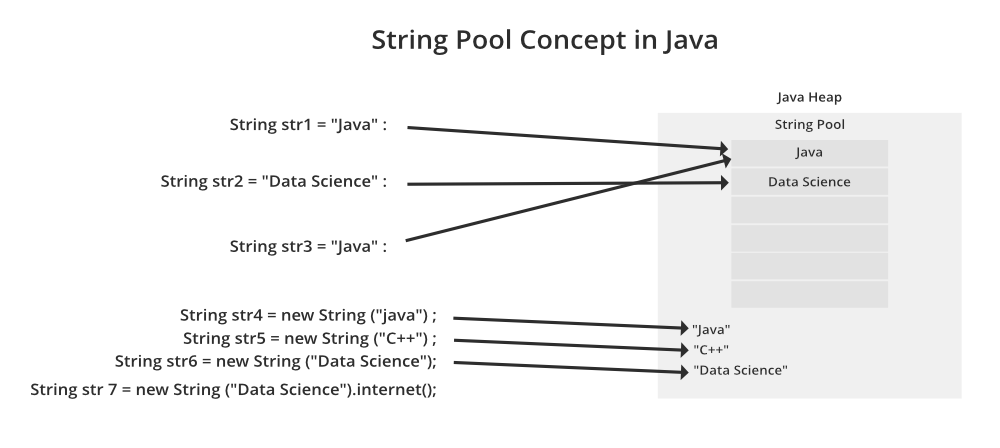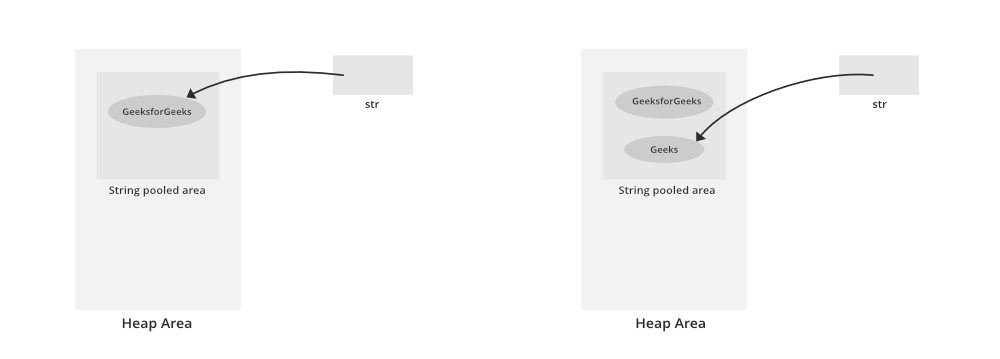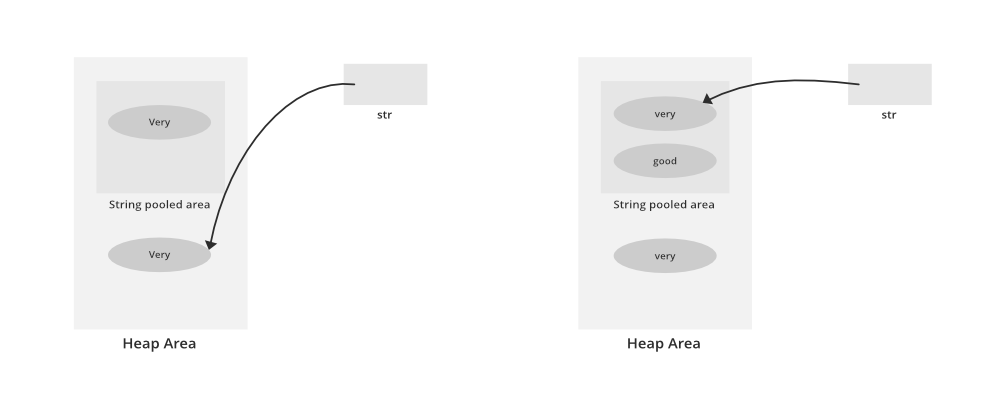Open In App

# How to Initialize and Compare Strings in Java?

As we all know String is immutable in java so do it gives birth to two ways of initialization as we do have the concept of String Pool in java.

Ways: Initializing Strings in Java

1. Direct initialization
2. Indirect initializationWay 1: Direct Initialization(String Constant)

In this method, a String constant object will be created in String pooled area which is inside the heap area in memory. As it is a constant, we can’t modify it, i.e. String class is immutable.

Illustration:

```String str = "GeeksForGeeks";

str = "geeks"; // This statement will make str
// point to new String constant("geeks")
// rather than modifying the previous
// String constant.```From the left media

`String str = "GeeksForGeeks"; `

From the right media above

`str = "geeks"; `

Note: If we again write str = “GeeksForGeeks” as next line, then it first check that if given String constant is present in String pooled area or not. If it present then str will point to it, otherwise creates a new String constant.

Way 2: Object Initialization (Dynamic)

In this method, a String object will be created in the heap area (not inside String pooled area as in the upper case). We can’t modify it(like in the upper case). Also with the same value, a String constant is also created in the String pooled area, but the variable will point to the String object in the heap area only.

Illustration:

```String str = new String("very");
str = "good";     ```

We can draw some conclusions out from them as follows:

In left from the below media

`String str = new String("very"); `

In right from the below media as follows:

`str = "good" `Now, this is a direct assignment, so a String constant with the value “good” is created in the String pooled area and str will point to that.

Note: If we again write str = new String(“very”), then it will create a new object with value “very”, rather than pointing to the available objects in heap area with same value.But if we write str = “very”, then it will point to String constant object with value “very”, present in String pooled area.

Methods: Comparing Strings and their References

1. equals() method: It compares values of string for equality. Return type is boolean. In almost all the situation you can use Objects.equals().
2. == operator: It compares references not values. Return type is boolean. == is used in rare situations where you know you’re dealing with interned strings.
3. compareTo() method: It compares values lexicographically and returns an integer value that describes if first string is less than, equal to, or greater than the second string. For example, if str1 and str2 are two string variables then refer below as follows:
• str1 == str2 : return 0
• str1 > str2 : return a positive value
• str1 < str2 : return a negative value

Note: The positive and negative values returned by compareTo method is the difference of first unmatched character in the two strings.

Implementation: We will be discussing how to compare to strings to justify the above said via below example

Example:

## Java

 `// Java program to Illustrate Comparison of Two Strings`` ` `// Main class``public` `class` `GFG {`` ` `    ``// Main driver method``    ``public` `static` `void` `main(String[] args)``    ``{``        ``// Custom input strings to compare``        ``String s1 = ``"Ram"``;``        ``String s2 = ``"Ram"``;``        ``String s5 = ``"Shyam"``;`` ` `        ``String s3 = ``new` `String(``"Ram"``);``        ``String s4 = ``new` `String(``"Ram"``);`` ` `        ``// Checking whether strings are equal or not``        ``// with help of equals() method``        ``System.out.println(``            ``" Comparing strings with equals:"``);``        ``System.out.println(s1.equals(s2));``        ``System.out.println(s1.equals(s3));``        ``System.out.println(s1.equals(s5));`` ` `        ``String nulls1 = ``null``;``        ``String nulls2 = ``null``;`` ` `        ``// NullPointerException will be throws if``        ``// we try to compare nulls strings``        ``// System.out.println(nulls1.equals(nulls2));`` ` `        ``// Comparing strings using == operator``        ``System.out.println(``" Comparing strings with ==:"``);``        ``System.out.println(s1 == s2);``        ``System.out.println(s1 == s3);``        ``System.out.println(s3 == s4);``        ``System.out.println(nulls1 == nulls2);`` ` `        ``// Comparing strings via compareTo() method``        ``System.out.println(``            ``" Comparing strings via compareTo() Method :"``);``        ``System.out.println(s1.compareTo(s3));``        ``System.out.println(s1.compareTo(s5));`` ` `        ``// NullPointerException if we try to compare strings``        ``// with usage of compareTo() method``        ``// System.out.println(nulls1.compareTo(nulls2));``    ``}``}`

Output

``` Comparing strings with equals:
true
true
false
Comparing strings with ==:
true
false
false
true
Comparing strings via compareTo() Method :
0
-1```